## Exercise 16.1 Page No: 255

Find the values of the letters in each of the following and give reasons for the steps involved.

1.Solution:

Say, A = 7 and we get,

7+5 = 12

In which one’s place is 2.

Therefore, A = 7

And putting 2 and carry over 1, we get

B = 6

Hence A = 7 and B = 6

2.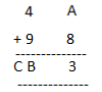Solution:

If A = 5 and we get,

8+5 = 13 in which ones place is 3.

Therefore, A = 5 and carry over 1 then

B = 4 and C = 1

Hence, A = 5, B = 4 and C = 1

3.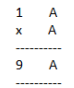Solution:

On putting A = 1, 2, 3, 4, 5, 6, 7 and so on and we get,

AxA = 6×6 = 36 in which ones place is 6.

Therefore, A = 6

4.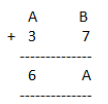Solution:

Here, we observe that B = 5 so that 7+5 =12

Putting 2 at ones place and carry over 1 and A = 2, we get

2+3+1 =6

Hence A = 2 and B =5

5.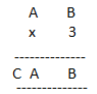Solution:

Here on putting B = 0, we get 0x3 = 0.

And A = 5, then 5×3 =15

A = 5 and C=1

Hence A = 5, B = 0 and C = 1

6.Solution:

On putting B = 0, we get 0x5 = 0 and A = 5, then 5×5 =25

A = 5, C = 2

Hence A = 5, B = 0 and C =2

7.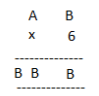Solution:

Here product of B and 6 must be same as ones place digit as B.

6×1 = 6, 6×2 = 12, 6×3 = 18, 6×4 =24

On putting B = 4, we get the ones digit 4 and remaining two B’s value should be44.

Therefore, for 6×7 = 42+2 =44

Hence A = 7 and B = 4

8.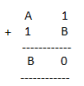Solution:

On putting B = 9, we get 9+1 = 10

Putting 0 at ones place and carry over 1, we get for A = 7

7+1+1 =9

Hence, A = 7 and B = 9

9.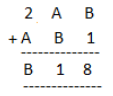Solution:

On putting B = 7, we get 7+1 =8

Now A = 4, then 4+7 =11

Putting 1 at tens place and carry over 1, we get

2+4+1 =7

Hence, A = 4 and B = 7

10.Solution:

Putting A = 8 and B = 1, we get

8+1 =9

Now, again we add2 + 8 =10

Tens place digit is ‘0’ and carry over 1. Now 1+6+1 = 8 =A

Hence A = 8 and B =1

## Exercise 16.2 Page No: 260

1. If 21y5 is a multiple of 9, where y is a digit, what is the value of y?

Solution:

Suppose 21y5 is a multiple of 9.

Therefore, according to the divisibility rule of 9, the sum of all the digits should be a multiple of 9.

That is, 2+1+y+5 = 8+y

Therefore, 8+y is a factor of 9.

This is possible when 8+y is any one of these numbers 0, 9, 18, 27, and so on

However, since y is a single digit number, this sum can be 9 only.

Therefore, the value of y should be 1 only i.e. 8+y = 8+1 = 9.

2. If 31z5 is a multiple of 9, where z is a digit, what is the value of z? You will find that there are two answers for the last problem. Why is this so?

Solution:

Since, 31z5 is a multiple of 9.

Therefore according to the divisibility rule of 9, the sum of all the digits should be a multiple of 9.

3+1+z+5 = 9+z

Therefore, 9+z is a multiple of 9

This is only possible when 9+z is any one of these numbers: 0, 9, 18, 27, and so on.

This implies, 9+0 = 9 and 9+9 = 18

Hence 0 and 9 are two possible answers.

3. If 24x is a multiple of 3, where x is a digit, what is the value of x?

(Since 24x is a multiple of 3, its sum of digits 6+x is a multiple of 3; so 6+x is one of these numbers: 0, 3, 6, 9, 12, 15, 18, … . But since x is a digit, it can only be that 6+x = 6 or 9 or 12 or 15. Therefore, x = 0 or 3 or 6 or 9. Thus, x can have any of four different values.)

Solution:

Let’s say, 24x is a multiple of 3.

Then, according to the divisibility rule of 3, the sum of all the digits should be a multiple of 3.

2+4+x = 6+x

So, 6+x is a multiple of 3, and 6+x is one of these numbers: 0, 3, 6, 9, 12, 15, 18 and so on.

Since, x is a digit, the value of x will be either 0 or 3 or 6 or 9, and the sum of the digits can be 6 or 9 or 12 or 15 respectively.

Thus, x can have any of the four different values: 0 or 3 or 6 or 9.

4. If 31z5 is a multiple of 3, where z is a digit, what might be the values of z?

Solution:

Since 31z5 is a multiple of 3.

Therefore according to the divisibility rule of 3, the sum of all the digits should be a multiple of 3.

That is, 3+1+z+5 = 9+z

Therefore, 9+z is a multiple of 3.

This is possible when the value of 9+z is any of the values: 0, 3, 6, 9, 12, 15, and so on.

At z = 0, 9+z = 9+0 = 9

At z = 3, 9+z = 9+3 = 12

At z = 6, 9+z = 9+6 = 15

At z = 9, 9+z = 9+9 = 18

The value of 9+z can be 9 or 12 or 15 or 18.

Hence 0, 3, 6 or 9 are four possible answers for z.

### NCERT Solutions for Class 8 Maths Chapter 16 Playing with Numbers

Class 8 NCERT exercise wise questions and answers are very important for students in order to develop logical and mental ability. Some of the important topics introduced in Solutions of Class 8 NCERT Maths are Numbers in General Form, Games with Numbers, Letters for Digits and Tests of Divisibility. In previous classes, students have studied various types of numbers, their properties and relationships among them. In this section, numbers are explored in more detail.

### NCERT Solutions for Class 8 Maths Chapter 16 Playing with Numbers

NCERT Class 8 Maths Chapter 16-Playing with Numbers, introduce Numbers in General Form, Games with Numbers and Divisibility test by some numbers: by 2, 3, 5, and 10.

The main topics covered in this chapter include:

 Exercise Topic 16.1 Introduction 16.2 Numbers in General Form 16.3 Games with Numbers 16.4 Letters for Digits 16.5 Tests of Divisibility

### Key Features of NCERT Solutions for Class 8 Maths Chapter 16 Playing with Numbers

1. These NCERT exercises are meticulously solved by subject experts.
2. Simple and easy language is used.
3. All questions are solved using a step-by-step approach.
4. Practise all the solved examples given and get exam ready.
5. These NCERT solutions are a valuable help to students in their assignments and competitive exams.

Disclaimer:

Dropped Topics – 16.1 Introduction, 16.2 Numbers in General Form, 16.3 Games with Numbers, 16.4 Letters for Digits and 16.5 Tests of Divisibility.